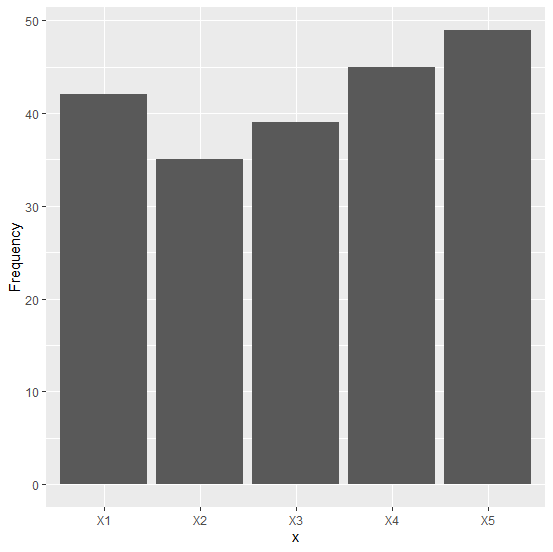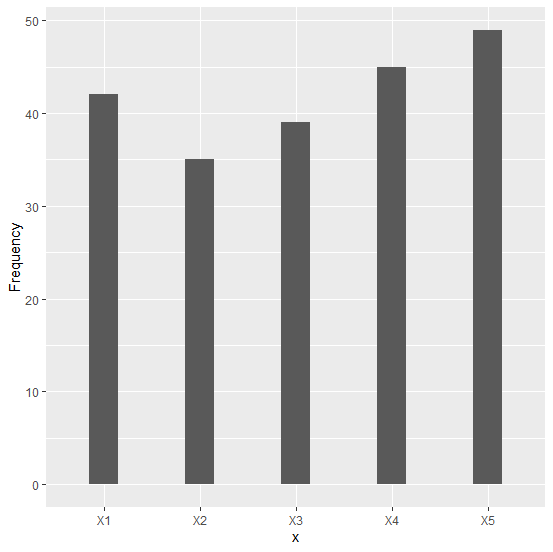# How to increase the space between bars of a bar plot using ggplot2 in R?

When a bar plot is created then the distance or space between bars is equal but sometimes the width of the bar is large, therefore, it becomes a little difficult to understand the difference between those bars especially in cases when the data values are not very much different from each other. To overcome this visualization problem, we can create a bar plot with some space between the bars and it can be done with the help of width argument of geom_bar in ggplot2.

## Example

Consider the below data frame −

x<-c("X1","X2","X3","X4","X5")
Frequency<-c(42,35,39,45,49)
df<-data.frame(x,Frequency)
df
x Frequency
1 X1 42
2 X2 35
3 X3 39
4 X4 45
5 X5 49
library(ggplot2)
ggplot(df,aes(x,Frequency))+geom_bar(stat='identity')

## OutputNow to increase the space between bars can be done as follows −

ggplot(df,aes(x,Frequency))+geom_bar(stat='identity',width=0.3)

## Output